## 4.1Boundary mesh

Sec. 3.2 describes the computational mesh used by the ﬁnite volume method. It identiﬁes a domain boundary by faces which are connected only to one cell. The boundary faces are grouped into patches, each under a unique name, corresponding to diﬀerent regions of the boundary. Boundary conditions are then applied to each patch to provide a representative solution to the case of interest.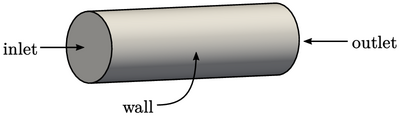In the example of ﬂow in a pipe, it would be logical to split the boundary into 3 patches in order to specify inﬂow, outﬂow and solid walls; and, inlet, outlet and wall would be logical names for these patches.

### Patch geometric data

The geometry of a patch is described using face data described in Sec. 3.3 , including:

• the face area vector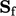, with area magnitude and direction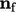;
• the face unit normal vector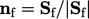;
• the face centre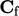.

The cell connected to each patch face is denoted by the subscript “P”, e.g. the cell centre is denoted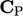.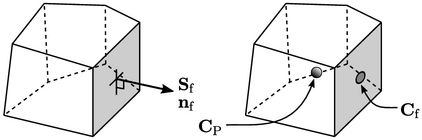### Patch deltas

Sec. 3.8 describes the “delta”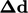for each face as the vector connecting the centres of its owner and neighbour cells.is deﬁned diﬀerently for a patch face since it has no neighbour cell.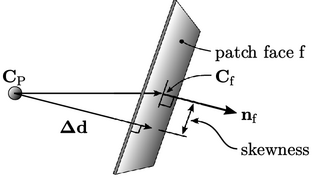The delta is deﬁned as the component of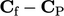in the face normal direction. The surface gradient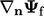is orthogonal to the face, which eliminates the error associated with non-orthogonality, at the expense of introducing a skewness error. Taking the inner product with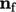gives the magnitude which is then multiplied byto assign the direction, i.e.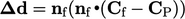(4.1)
The delta coeﬃcients are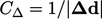, as deﬁned in Sec. 3.8 . The delta coeﬃcient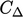, representing “inverse distance”, is a critical parameter in the discretisation of boundary conditions.
Notes on CFD: General Principles - 4.1 Boundary mesh